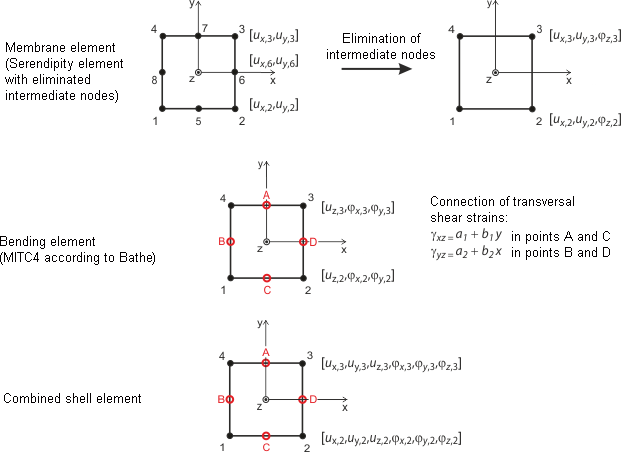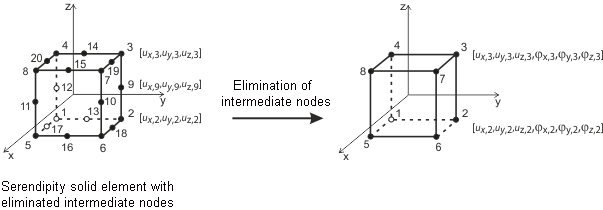# RFEM – Online Manual

Online manuals, introductory examples, tutorials, and other documentation.

# 7.2.1 Basics of Finite Elements in RFEM

### Basics of Finite Elements in RFEM

1D elements

For member elements, it is assumed that the cross-section remains planar when deforming. 1D member elements are used to display beams, trusses, ribs, cables, and rigid couplings. In total, a 1D member element has 12 degrees of freedom: six at the start and six at the end of the element. They refer to displacements (ux, uy, uz) and rotations (φx, φy, φz). When calculating structural data linearly, tension, compression, and torsion are expressed as linear functions of the member axis x, independent of bending and shear. They are approximated by a 3rd order polynomial in x, including influence of shearing stresses resulting from the shear forces Vy and Vz. The stiffness matrix KL(12, 12) describes the linear behavior of the 1D elements. The mutual interaction between axial force and bending in case of geometrically nonlinear problems is expressed in the stiffness matrix KNL(12, 12). You can find more information in  and .

For calculations according to the large deformation analysis, it is recommended to use an FE mesh refinement of lines (see Chapter 4.23) so that results can be calculated accurately.

2D elements

Usually, quadrangle elements are used as 2D elements. The mesh generator adds triangular elements where required.

The degrees of freedom in corner nodes of quadrangle and triangular elements are the same as for 1D elements: degrees of freedom of displacement (ux, uy, uz) and rotation (φx, φy, φz). In this way, the compatibility of 1D and 2D elements in nodes is guaranteed. The parameters are defined in the planar local coordinate system of elements and are converted into the global coordinate system when creating the global stiffness matrix.Figure 7.8 Shell elements of RFEM (quadrangle)

The planar shell elements are based on the Mindlin/Reissner theory. Figure 7.8 shows the approaches of elements graphically. To ensure a direct coupling with member elements, a square approach in the shell plane (ux, uy) is chosen. By eliminating the intermediate nodes, a four-node element with an additional degree of freedom φx is created. This allows for wall elements to be directly coupled with beam elements. Based on a mixed interpolation of transversal deformations, cross-section rotations, and transversal shear strains, the MITC4 elements (Mixed Interpolation of Tensorial Components) as presented by Bathe and Dvorkin  are also applied.

Presently, member elements are considered by directly solving the differential equation according to the second-order analysis. Considering drilling effects is not possible when using the Saint Venant torsion.

The analysis of membranes is based on the principles of Bergan , , . For triangular elements, for example, the basic functions are divided into three rigid-body deformations, three constant conditions of strain, and three special linear gradients of stress and strain. Within an element, the deformation field is quadratic and the stress field is linear. The element stiffness matrix KL is subsequently transformed into nine collective parameters of the types ux, uy, φz. The components of this matrix are added to the overall stiffness matrix (18, 18) together with the components that cause bending and shear effects. This matrix is the result of the Lynn/Dhillon concept. Then, so-called Mindlin plates are applied, which means that plates with distinct shear distortion are analyzed according to Timoshenko. Thus, RFEM is able to find the correct solution for both thick and thin plates (Navier plates).

In case of geometrically nonlinear problems, the above-mentioned split of the stress-strain condition into a planar state and bending with shear is not possible. The mutual influences of these states are considered in the matrix KNL. RFEM uses a rather simple but effective type of the KNL matrix that is based on the approaches of Zienkiewicz . The square component ε2 of the Green/Lagrange strain tensor ε = ε1 + ε2 is applied. A linear distribution of uz(x, y) of the planar stress condition and linear distributions of ux(x, y) and uy(x, y) of the interaction with bending is assumed. This assumption is possible, because the main effect of the interaction depends on the first derivation of the differential equation, and because the influence of components of a higher order decreases quickly with the division into smaller elements. The correctness of this procedure has been proven in several numerical analyses.

For shell elements, the thickness of the elements must be considerably smaller than their expansion. If this is not the case, it is recommended to model objects as solids. Furthermore, when using shell elements, a point-by-point introduction of torsional stresses should be avoided: The rotational degree of freedom about the surface normal reacts very sensitively.

3D elements

The following 3D elements are implemented in RFEM: tetrahedron, pentahedron (prism, pyramid), and hexahedron. For detailed information about applied elements and matrices, see . Corresponding documentation can be requested from Dlubal Software.Figure 7.9 Solid element (hexahedron)

Generally, all rotational degrees of freedom must be regarded as critical for solids. As the deformation of a solid is solely determined from the displacement vectors, the rotation of a mesh node, for example due to singularly introduced torsion, does not affect the deformation within the solid.

Literatur
  Vladimír Kolář et al. Bemessung von zwei- und dreidimensionalen Strukturen mit FEM. Springer-Verlag, New York / Wien, 1975. Chapters 1 (1D element) and 6 (variational principle)  Vladimír Kolář and Ivan Němec. Finite Element Analysis of Structures. United Nations Development Program, Economic Com. for Europe, Workshop on CAD Techniques, Prague - Geneva, 1984.  Eduardo N. Dvorkin and Klaus-Jürgen Bathe. A continuum mechanics based four-node shell element for general non-linear analysis. Engineering Computations, 1, 1984.  P. G. Bergan. Finite Elements Based on Energy Orthogonal Functions. International Journal for Numerical Methods in Engineering, 15, 1980.  P. G. Bergan and M. K. Nygård. Finite Elements With Increased Freedom in Choosing Shape Functions. International Journal for Numerical Methods in Engineering, 20, 1984.  P. G. Bergan and Carlos A. Felippa. A Triangular Membrane Element With Rotational Degrees of Freedom. Computer Methods in Applied Mechanics and Engineering, 50, 1985.  Olgierd Cecil Zienkiewicz. The Finite Element Method in Engineering Science. Mc Graw-Hill, London edition = 3, 1979. Chapter 18 - 19 (Nonlinear Problems).  I. Sevčík. 3D Finite Elements with Rotational Degrees of Freedom. FEM Consulting s.r.o, Brno.